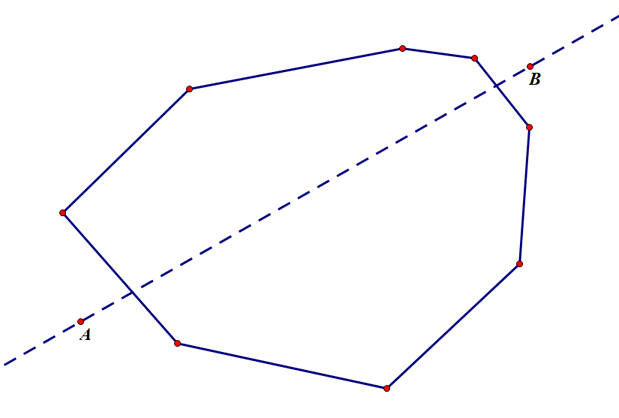# 4554. Cake

XiaoNie wants to share birthday cake with friends. The cake is a convex polygon on a plane. Its $n$ points are given by clockwise.

Give two points, XiaoNie will cut the cake along the straight line determined by the two points, dividing the cake into two parts. XiaoNie will eat the smaller part. If the areas of the two parts are equal, XiaoNie will choose any one of them. Now we want to know the area of cake eaten by XiaoNie.### 输入格式

The first line, a postive integer $n$ , means the number of the cake points.

The following $n$ lines, the $i$ th line is two integers $x_i, y_i$ , means the $i$ th point of the cake.

The last line is four integers $x_a,y_a,x_b,y_b$ , means cutting the cake along the straight line determined by $(x_a,y_a)$ and $(x_b,y_b)$ .

### 输出格式

One line, one real number, means the area of the smaller part of cake.

Your answer will be considered correct if it is within $10^{-5}$ of the standard answer in absolute terms.

### 样例

Input
3
0 2
2 0
0 0
0 0 1 1

Output
1.00000

Input
4
2 2
7 2
5 0
0 0
3 1 3 3

Output
4.00000


### 提示

$3\le n \le 100$

$-10^5\le x_i,y_i,x_a,y_a,x_b,y_b\le 10^5$

Data guarantees the last two points can determine a straight line and the straight line divides the cake into two parts.

Sample 1 Explanation:

The cake is a triangle of area 2.0, XiaoNie divided it into two small triangles of area 1.0, so the answer is 1.0.

48 人解决，63 人已尝试。

56 份提交通过，共有 219 份提交。

4.3 EMB 奖励。